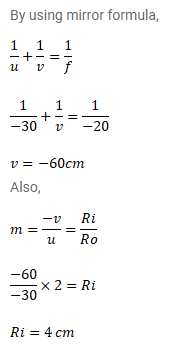# A particle goes in a circle of radiusQuestion:

A particle goes in a circle of radius $2.0 \mathrm{~cm}$. A concave mirror of focal length $20 \mathrm{~cm}$ is placed with its principal axis passing through the centre of the circle and perpendicular to its plane. The distance between the pole of the mirror and the center of the circle is $30 \mathrm{~cm}$. Calculate the radius of the circle formed by the image.

Solution: